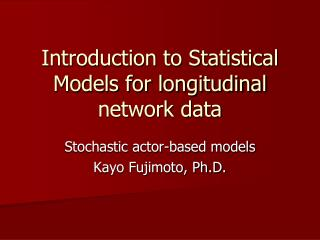Download PresentationIntroduction to Statistical Models for longitudinal network dataIntroduction to Statistical Models for longitudinal network data - PowerPoint PPT Presentation

Download PresentationIntroduction to Statistical Models for longitudinal network data
An Image/Link below is provided (as is) to download presentation

Download Policy: Content on the Website is provided to you AS IS for your information and personal use and may not be sold / licensed / shared on other websites without getting consent from its author. While downloading, if for some reason you are not able to download a presentation, the publisher may have deleted the file from their server.

- - - - - - - - - - - - - - - - - - - - - - - - - - - E N D - - - - - - - - - - - - - - - - - - - - - - - - - - -
Presentation Transcript

1. Introduction to Statistical Models for longitudinal network data Stochastic actor-based models Kayo Fujimoto, Ph.D.

2. Stochastic actor-based model(Snijders 2001, 2005) • Actor-orientedmodeling • Methodological individualism • Modeled as a consequence of actors: • Making new choices • Withdrawing existing choice • Functions that actors try to maximize • Continuous-time Markov chain models • Simulation models

3. Dependent variable • Changing relation network • Number of changed ties between consecutive observations

4. Independent Variables • Change in the network (DV) is modeled as the stochastic result of network effects (such as reciprocity, transitivity, etc.) and covariates

5. Model assumptions • Full knowledge of the present network • All actors control their outgoing relations • A specific actor i has the opportunity to change their relations one at a time at stochastic moment t at a rateρm • Model specification: changes of single relations

6. Three types of effects • Rate function effects • Models the speed by which the DV changes • Objective function effects • Models the actors’satisfaction with their local network configuration • Endowment function effects • Model the loss of satisfaction incurred when existing network ties are dissolved

7. Objective function effect • Determines probabilistically the tie changes made by the actors • Defined as a function of the network • regarded from the perspective of the focus actor • Depends on parameters • estimated from the data

8. Objective function • Network evaluation function for actor i • Degree of satisfaction for each actor i in relation x

9. Structural effects (examples) • Outdegree effect (density effect) • Reciprocity effect • Triad effect (transitivity, cycle, balance etc.)

10. Examples –transitive triplets effect

11. Covariate effects (V) • Covariate-ego effect (sender effect) • Whether actors with higher V values tend to nominate more friends and hence have a higher outdegree • Covariate-alter effect (receiver effect) • Whether actors with higher V values tend to be nominated by more others and hence have higher indegrees • Covariate-similarity effect (homophily) • Whether ties tend to occur more often between actors with similar values o n V

12. Covariate effects (examples) • Covariate-ego effect (covariate-related activity) • Covariate-alter effect (covariate-related popularity) • Same covariate effect (homophily)

13. Objective function • Actor i chooses alter j that maximize the value of her objective function fi(x) • Plus random element (Gumbel dist’n) • The part of the actor’s preference that is not represented by the systematic component of fi(x)

14. Model Parameters • Estimated from observed data • Stochastic simulation models • MCMC algorithm • Approximate the solution of the Method of Moment

15. Estimation in SIENA • Choose statistics • Obtain parameters such that the expected values of the statistics are equal to the observed values • Expected values are approximated as the averages over a lot of simulated network • Observed values are calculated from the dataset (target values)

16. Estimation in SIENA • Iterative stochastic simulation algorithm • In phase 1: the sensitivity of the statistics to the parameters is determined • In phase 2: provisional parameter values are updated • Simulate a network based on provisional parameter values • Compute the deviations between these simulated statistics and target values • Update parameter values • In phase 3: the final results of phase 2 is used and checked if the average statistic of many simulated networks are close to the targeted values • t statistics for deviations from targets

17. Longitudinal network dynamic models • Actor-oriented models of Snijders and colleagues • Assumption: network change driven by actors seeking to optimize particular structural positions • Longitudinal versions of ERGM (tie-based version of the model) • Assumption: network change driven by change in tie variables (particular social neighborhood of other ties)

18. References • Snijders, T.A.B.(2001). The statistical evaluation of social network dynamics, Sociological Methodology • Snijders, T.A.B.(2005). Models for longitudinal network data, chapter 11 in Carrington, P., Scott, J, Wasserman S (eds), models and methods in social network analysis. New York: Cambridge University Press.